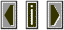Ohm's Law & related equalitiesdefinitions:

 E is electrical voltage potential in volts.  I is the flow of electrons in amperes current flows from the point of highest potential (negative) to the point of lowest potential (positive)  R is resistance to the flow of current in ohms.  Z is impedance to the flow of alternating current in ohms.  P is electrical power to do work in watts

 equations:

 I=E/R R=E/I E=I*R  I=E/Z Z=E/I E=I*Z  P=E*I  P=E**2/R(or Z)  P=I**2*R(or Z)  E = P/I  I*R  I*Z  square root of (P*R)  square root of (P*Z)  I = P/E  E/R  E/Z  square root of (P/R)  square root of (P/Z)  R = E/I Z = E/I P/I**2 P/I**2 E**2/P E**2/P P = E*I R*(I**2) Z*(I**2)  E**2/R  E**2/Z